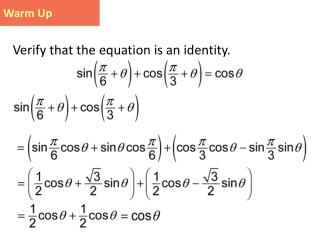Download PresentationWarm Up

Warm Up - PowerPoint PPT Presentation

Warm Up. Verify that the equation is an identity. Trig Game Plan Date : 12/09/13. Sum and Difference Identities for Tangent. We can use the cosine sum and difference identities to derive similar identities for sine and tangent. Fundamental identity. Sum identities.I am the owner, or an agent authorized to act on behalf of the owner, of the copyrighted work described.
Download PresentationWarm Up

Download Policy: Content on the Website is provided to you AS IS for your information and personal use and may not be sold / licensed / shared on other websites without getting consent from its author.While downloading, if for some reason you are not able to download a presentation, the publisher may have deleted the file from their server.

- - - - - - - - - - - - - - - - - - - - - - - - - - E N D - - - - - - - - - - - - - - - - - - - - - - - - - -
Presentation Transcript
1. Warm Up Verify that the equation is an identity.

2. Trig Game Plan Date: 12/09/13

3. Sum and Difference Identities for Tangent We can use the cosine sum and difference identities to derive similar identities for sine and tangent. Fundamental identity Sum identities Multiply numerator and denominator by 1.

4. Sum and Difference Identities for Tangent Multiply. Simplify. Fundamental identity

5. Sum and Difference Identities for Tangent Replace B with –B and use the fact that tan(–B) to obtain the identity for the tangent of the difference of two angles.

6. Tangent of a Sum or Difference tan ( A ± B ) = tan A ± tan B 1 tan A tan B ± -

7. WRITING FUNCTIONS AS EXPRESSIONS INVOLVING FUNCTIONS OF θ Example Write each function as an expression involving functions of θ. (a) (b)

8. FINDING EXACT SINE AND TANGENT FUNCTION VALUES Example 1(d) Find the exact value of

9. FINDING EXACT SINE AND TANGENT FUNCTION VALUES Example 1(e) 5.4 Example 1 Finding Exact Sine and Tangent Function Values (cont.) (b)

10. FINDING EXACT SINE AND TANGENT FUNCTION VALUES Example 1(g) 5.4 Example 1 Finding Exact Sine and Tangent Function Values (cont.) (c)

11. VERIFYING AN IDENTITY USING SUM AND DIFFERENCE IDENTITIES Example 4a Combine the fractions. Verify that the equation is an identity.

12. VERIFYING AN IDENTITY USING SUM AND DIFFERENCE IDENTITIES Example 4a 5.4 Example 4 Verifying an Identity Using Sum and Difference Identities (cont.) Expand the terms. Combine terms. Factor. So, the identity is verified.